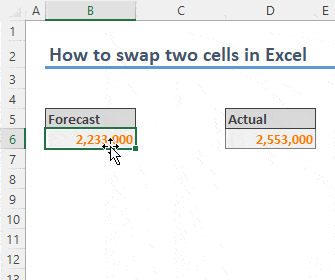Although swapping two cells sounds easy, it may become complicated what you want to be swapped or not. In this article, we are going to show how to swap two cells in Excel for several scenarios.

Let's start with the simplest and probably the one you will use most. Adjoining cells can be swapped easily by dragging and dropping while pressing the Shift Key.

1. Select one of the cells.
2. Press and hold the Shift key on your keyboard.
3. Hover the mouse cursor on the edges so you can see the 4-direction arrow ✥.
4. Click and move the pointer to the opposite side of the other cell.

You can use this technique to swap two cells in all directions. Also, this works on ranges as well.At the end, this approach is identical with the Cut & Insert Cut Cells (you can see this option on the right-click menu after cutting a cell or range) move set. Thus, you can use either Ctrl + + or Ctrl + Shift + = combinations after cutting, rather than moving with the mouse.

## Swapping two nonadjacent cells manually

To swap nonadjacent cells, you need a helper cell. The logic is simple:

1. Move one of the cells into an empty cell.
2. The other cell goes to the first cell's location.
3. Finally, move the helper cell into the second's location.## Swapping cells with a VBA macro

Almost all actions can be mimicked by VBA macros. However, swapping two cells is not one of them. Do not get me wrong, the above approaches are so primitive that can be replicated by a VBA macro easily. However, you cannot store a cell with entire contents and properties into a variable on the fly and populate it in another location.

Alternatively, you can use the VBA to swap two cells' contents or formulas in them easily. You can copy and paste the following macro to in a module in your workbook to start using right away.

```Sub SwapTwoCells()
Dim temp As String

If Selection.Cells.Count = 2 Then
With Selection
temp = .Cells(1).Formula ' Copy the formula
If .Areas.Count = 2 Then ' In case cells are selected using Ctrl key
.Areas(1).Formula = .Areas(2).Formula 'Swap formulas
.Areas(2).Formula = temp
Else ' In case adjacent cells are selected
.Cells(1).Formula = .Cells(2).Formula 'Swap formulas
.Cells(2).Formula = temp
End If
End With
Else
MsgBox "Please select two cells to swap" , vbCritical
End If
End Sub```

If you are new to VBA and macros, please refer How to create a macro in Excel for a quick guide.